## Arduino Setup and Installation

The TCLab works with MATLAB, Python, GNU Octave, Java, and any other software that can send and receive commands from a serial USB connection.

pip install tclab

The tclab package can also be installed from a Python script with the following code.

module='tclab' # for Arduino Leonardo
try:
from pip import main as pipmain
except:
from pip._internal import main as pipmain
pipmain(['install',module])

The Arduino Uno can be switched between MATLAB and Python compatibility by loading the appropriate firmware. When loading the Python firmware, select either Arduino UNO (2014-2017 versions) or Arduino Leonardo (2018+ TCLab version). The instructions in the video show how to load the firmware for an Arduino UNO or Arduino Hduino (green bottom). Select board type Arduino Leonardo for the newer version (white bottom). The board option should be changed to Arduino Leonardo if the back of the TCLab is white (not green).

The temperature control lab is pre-loaded with Python firmware. A first test is to send a command to the Arduino Uno to turn on the LED and adjust the LED level. The file tclab.py (in Python folder or pip installed) or tclab.m (in MATLAB folder) should be present in the search path or the run directory. The following scripts turn on the LED for 1 second.

Python

import tclab
import time
# Connect to Arduino
a = tclab.TCLab()
print('LED On')
a.LED(100)
# Pause for 1 second
time.sleep(1.0)
print('LED Off')
a.LED(0)
a.close()

MATLAB

clear all
% include tclab.m for initialization
tclab;
disp('LED on')
led(1)
% Pause for 1 second
pause(1.0);
disp('LED off')
led(0)

Once the LED test is successful, the heaters and temperature sensors can be tested. The top barrel jack powers the transistor heaters and should be plugged into a wall socket with the 5V power supply or else plugged into a second USB port on the computer. The following are minimal scripts to test the heaters and temperature sensors.

Python

import tclab
import numpy as np
import time
# Connect to Arduino
a = tclab.TCLab()
# Temperatures
print('Temperatures')
print('Temperature 1: ' + str(a.T1) + ' degC')
print('Temperature 2: ' + str(a.T2) + ' degC')
# Turn LED on
print('LED On')
a.LED(100)
# Turn on Heaters (0-100%)
print('Turn On Heaters (Q1=90%, Q2=80%)')
a.Q1(90.0)
a.Q2(80.0)
# Sleep (sec)
time.sleep(30.0)
# Turn Off Heaters
print('Turn Off Heaters')
a.Q1(0.0)
a.Q2(0.0)
# Temperatures
print('Temperatures')
print('Temperature 1: ' + str(a.T1) + ' degC')
print('Temperature 2: ' + str(a.T2) + ' degC')
a.close()

MATLAB

clear all
% include tclab.m for initialization
tclab;
% Test device
disp(['Temperature 1: ' num2str(T1C()) ' degC'])
disp(['Temperature 2: ' num2str(T2C()) ' degC'])
disp('Turn on Heater 1 to 30%, Heater 2 to 60%')
h1(30);  h2(60);
disp('Turn on LED for 30 seconds')
led(1);   % ON
pause(30.0);
led(0); % OFF
disp('Turn off Heaters')
h1(0);  h2(0);
disp(['Temperature 1: ' num2str(T1C()) ' degC'])
disp(['Temperature 2: ' num2str(T2C()) ' degC'])
disp('Temperature Test Complete')

The TCLab Simulink interface gives control over the heaters and the ability to read the current temperatures. The TCLab is a block in a Simulink model that may include manual adjustments, PID control, Model Predictive Control, or other forms of control and estimation.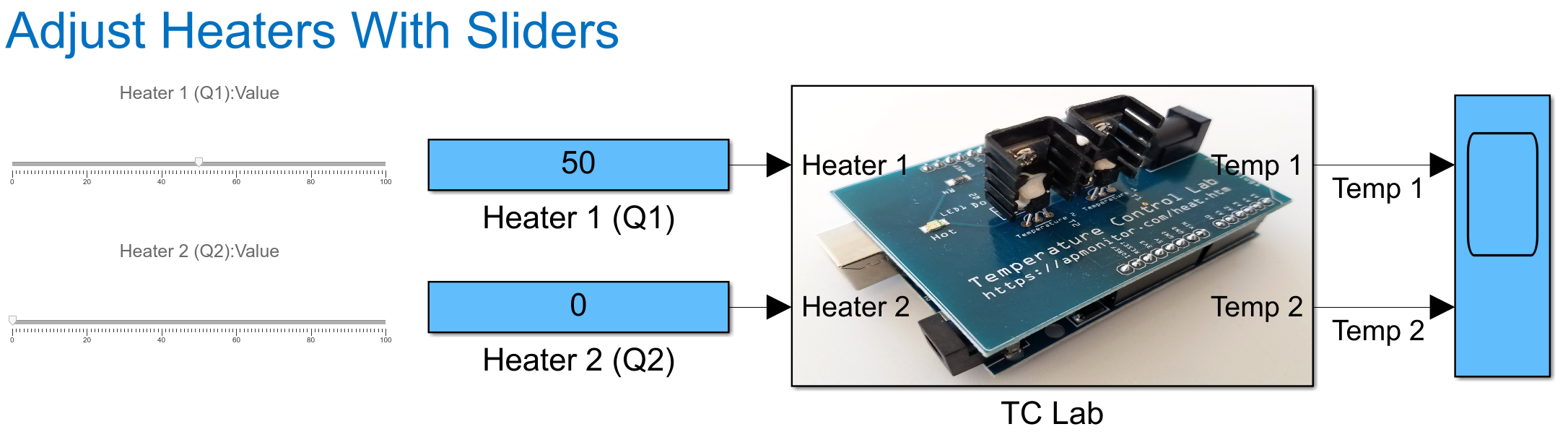TCLab Digital Twin

If no hardware is available, use TCLabModel() in the place of TCLab() in Python. The emulator (digital twin) can be run faster than real-time as shown below with Python:

import tclab
import numpy as np

tclab_hardware = False
if tclab_hardware:
mlab = tclab.TCLab      # Physical hardware
else:
speedup = 100           # Emulator (digital twin) speed-up
mlab = tclab.setup(connected=False, speedup=speedup)

n = 500
tm = np.linspace(0,2*n,n+1)

# Connect to TCLab
with mlab() as lab:
# set heater values
lab.Q1(70)
lab.Q2(20)
for t in tclab.clock(tm[-1]+1, 2):
print('Time: ' + str(t) + \
' T1: ' + str(round(lab.T1,2)) + \
' T2: ' + str(round(lab.T2,2)))

Change tclab_hardware from False to True to use the physical hardware.

For MATLAB, use the Simulink model Simulink\Single_Heater\arduino_lab.slx in the TCLab File Download archive (also available from the Download TCLab Files button on the Temperature Control Lab page).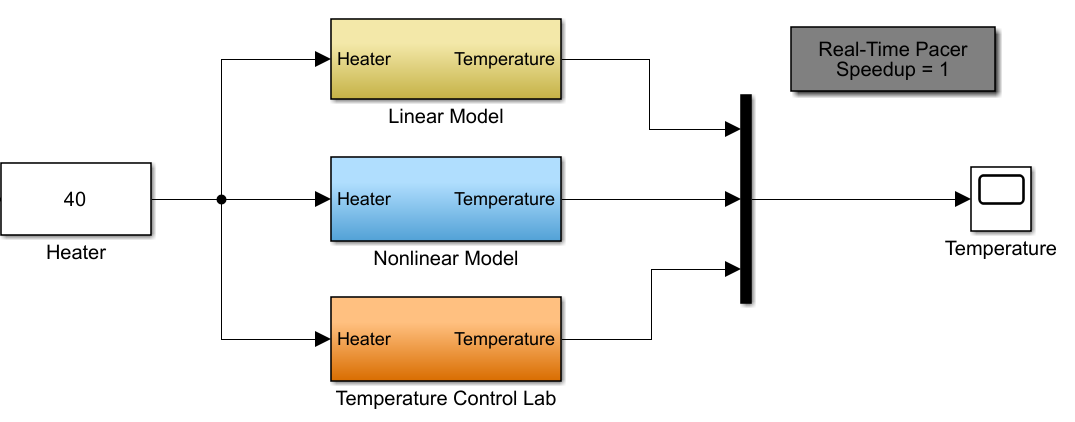Linear and nonlinear models are available with a speedup option. Remove the third block Temperature Control Lab (orange block) to run only the digital twins (linear and nonlinear simulators) when the TCLab is not connected.

#### Frequently Asked Questions

• Q: There is an error with ImportError: cannot import name 'Iterable' from 'collections' (C:\Users\Username\AppData\Local\Programs\ Python\Python310\lib\collections\__init__.py). How can this be fixed for Python 3.10+?
• A: The authors of the tclab package need to release an updated version for pip install on pypi.org. Until that update is made, edit the historian.py file (path is in the error message) with a text editor and change from collections import Iterable to:
#from collections import Iterable
from collections.abc import Iterable
Another way to resolve this issue is to get the latest unreleased version, install directly from GitHub.
pip install --upgrade https://github.com/jckantor/TCLab/archive/master.zip
Alternatively, returning to Python 3.9 also resolves the error.

• Q: What versions of MATLAB are supported?
• A: The TCLab uses an Arduino Leonardo. MATLAB 2015a+ is required. See the list from Mathworks on Arduino compatibility. The older TCLab version used an Arduino UNO with MATLAB 2014a+ compatibility.

• Q: Device isn't showing up as a device on my computer. What can I do to connect?
• A: First, verify that the cables are attached correctly.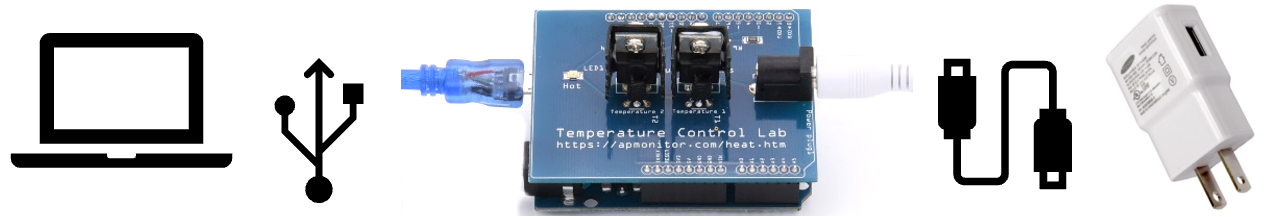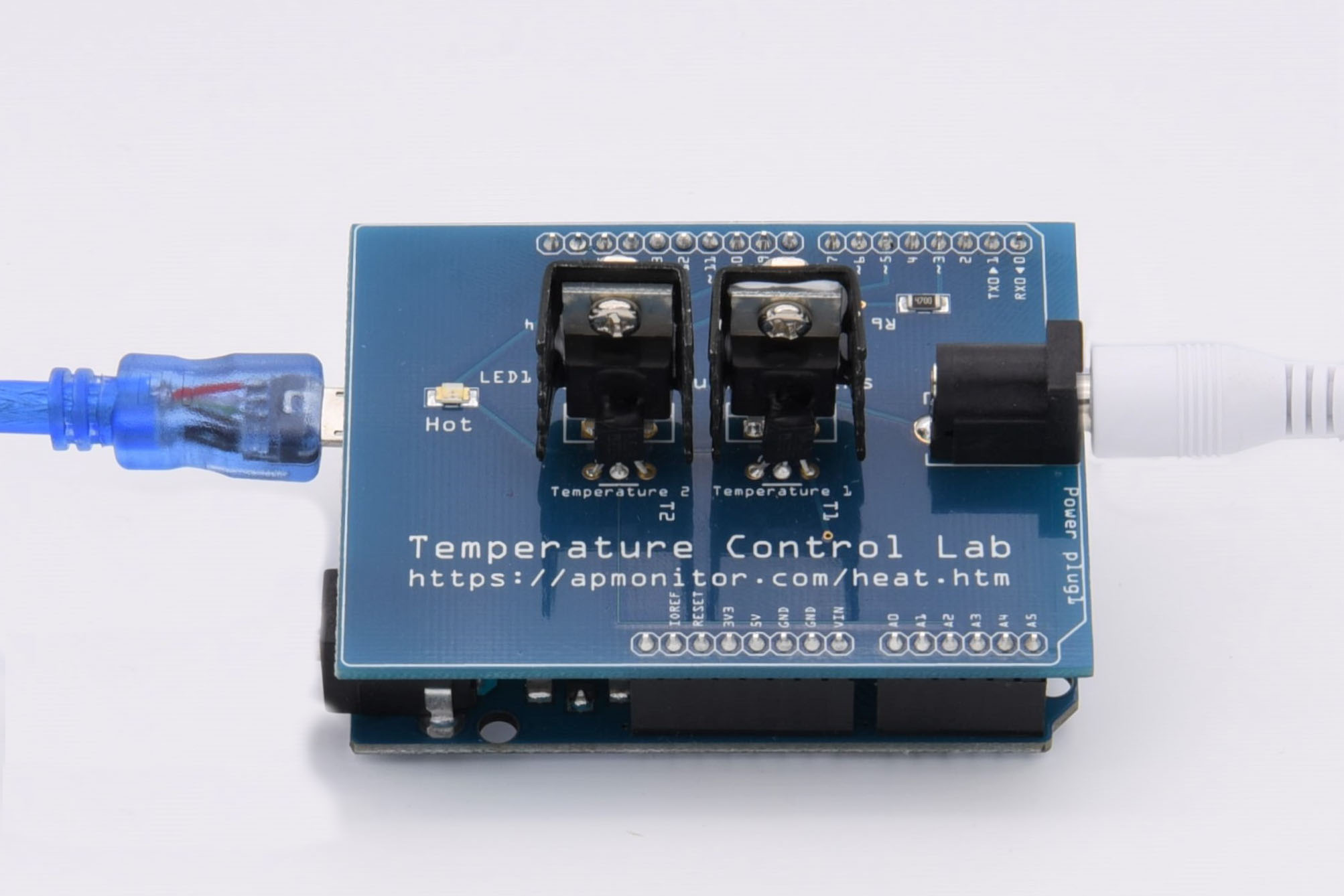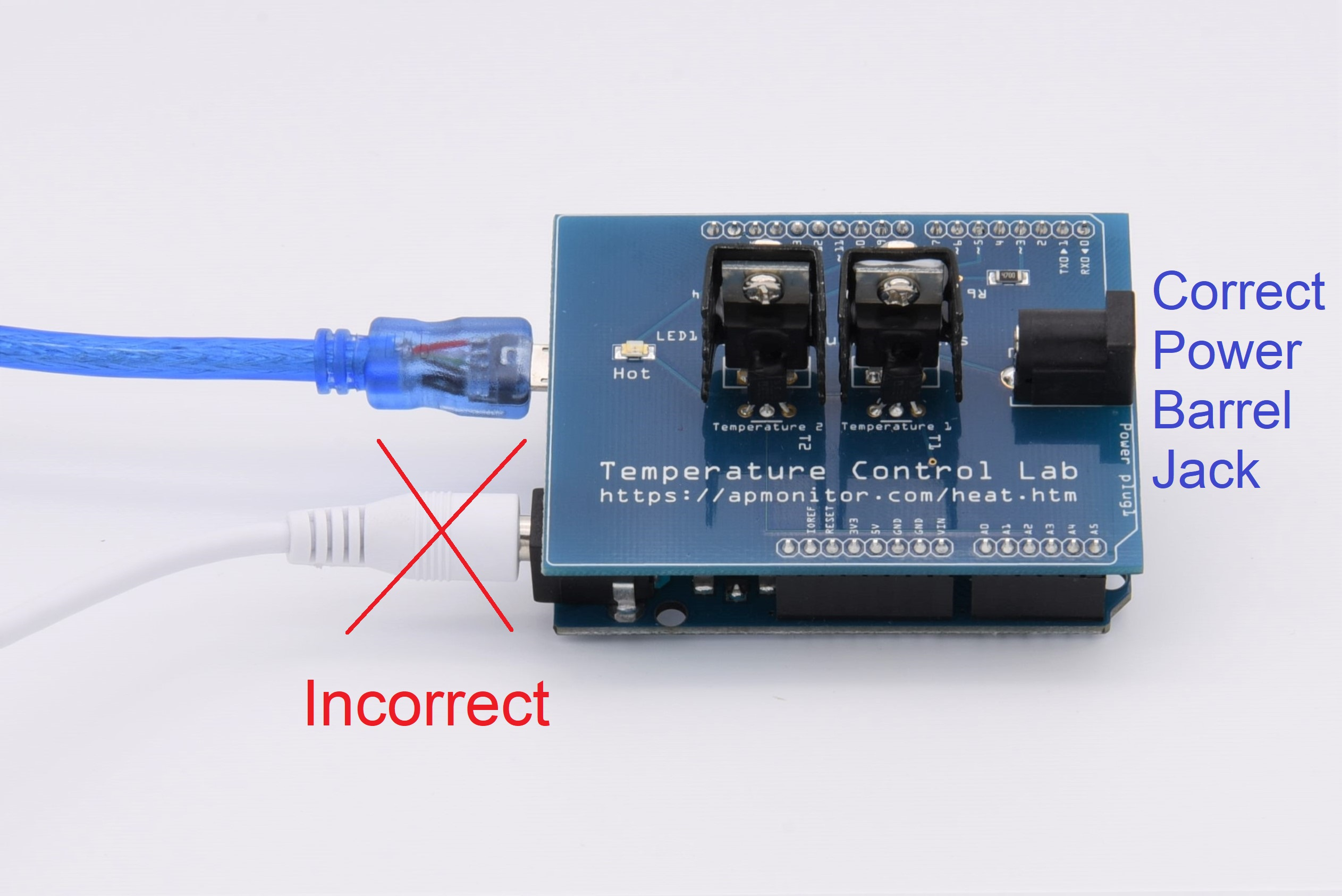There are two USB cables. One USB cable is for the top barrel jack to give power to the heaters. The other USB cable connects directly to a computer and gives power to the Arduino.Only the blue cable is required for the purpose of testing the communication between the Arduino and computer. The heater power cable can be attached later.The Arduino should now appear in the Device Manager (for Windows). Take note of the COM serial port assigned. In the example below, the COM port is COM3.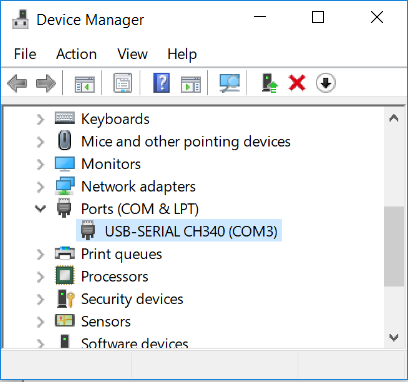For Linux and MacOS, you can see the device with a terminal and the following command:
ls /dev/*.
For Linux, give write permissions to the TCLab USB port.
sudo chmod a+rw /dev/ttyACM0
Change the ttyACM0 part depending on the USB port. To make this change permanent, add the command to the ~/.bashrc or ~/.zshrc file.
For MacOS, you may need to install drivers (only for Arduino UNO R3 pre-2018, not for Arduino Leonardo that is used after 2018). After the driver is installed, search for /dev/tty.usbserial* or /dev/cn.* (on MacOS) and /dev/ttyUSB* or /dev/ttyACM* (on Linux). No driver is required for the Arduino Leonardo base (Year 2018+ TCLab models).
For Windows 7, you will need to install driver from the CH340G / CH34G / CH34X chipset manufacturer. Windows 10 has the correct drivers and no driver installation is required.

• Q: How do I fix an error that the package serial could not be found?
• A: The package pyserial is missing. The tclab.py package attempts to install it automatically with pip.
module='pyserial'
try:
from pip import main as pipmain
except:
from pip._internal import main as pipmain
pipmain(['install',module])

Pyserial can also be installed from the command line with conda. There is more information on installing Python and managing packages here. You may need to run pip from the command line with administrative privileges.

pip install pyserial

• Q: How can I resolve an error that access is denied when attempting to connect to the Arduino?
• A: Reset the Arduino by either unplugging the USB connection and reconnecting or else press the red reset button. This should reset the Arduino device and allow it to connect again. This happens when there was an abnormal termination of the program and the serial connection is not closed.

• Q: How can I connect to the lab with Python?
• A: The tclab.py package automatically identifies the serial port (such as COM3) and attempts a connection. The temperature control labs are automatically loaded with tclab.ino (in the tclab folder) for Python. The Python tclab.ino program is recompiled and downloaded to the Arduino if the firmware is changed. The firmware is compiled and downloaded to the Arduino device with the Arduino software. The temperature control lab is pre-loaded with either Python or MATLAB firmware. See answer below if port is not found. No firmware changes should be required.

• Q: How do I fix an error that the port is not found for the serial connection?
• A: The tclab software automatically tries to find the USB serial port for connection. In some cases, the software is unable to find the correct port and tclab.py may need to be updated to select the correct port.
• For Windows:
• For MacOS:
• For Linux
• Open terminal and type: ls /dev/tty*
• Search for /dev/ttyUSB* or /dev/ttyACM*

• Q: How can I connect to the lab with MATLAB?
• A: The Arduino support package can be added to MATLAB. Once installed, the command a=arduino (for the Leonardo Arduino) or a = arduino('COM3','Uno') (for the Arduino UNO) loads the MATLAB Arduino drivers when MATLAB connects for the first time. Afterwards, only a = arduino is needed to establish a connection. This is built into the tclab.m file for MATLAB and Simulink.

• Q: The temperature is reading but the heaters don't seem to have an effect. How can I ensure that power is being supplied to the heaters?
• A: A USB to barrel jack cord provide power to the transistor heaters. Ensure that the barrel jack (round) connection is inserted into the top slot labeled Power plug1 and not into the barrel jack slot on the Arduino board. The USB power connection can also be plugged into a second computer USB port to provide power to the transistors although the temperature sensors may experience more random variation due to the pulse width modulation of the heaters. If the heaters operate successfully with the computer USB connection but not with the power supply then the power supply may have an internal trigger to shutoff if there is too much current draw, especially when both heaters are on at 100%.

• Q: How can I display real-time plots in Spyder (Python)?
• A: In the Spyder IDE menu bar select Tools, Preferences, IPython Console, Graphics, Backend=Automatic. This will allow real-time plots to display as a new window instead of inline in the IPython console.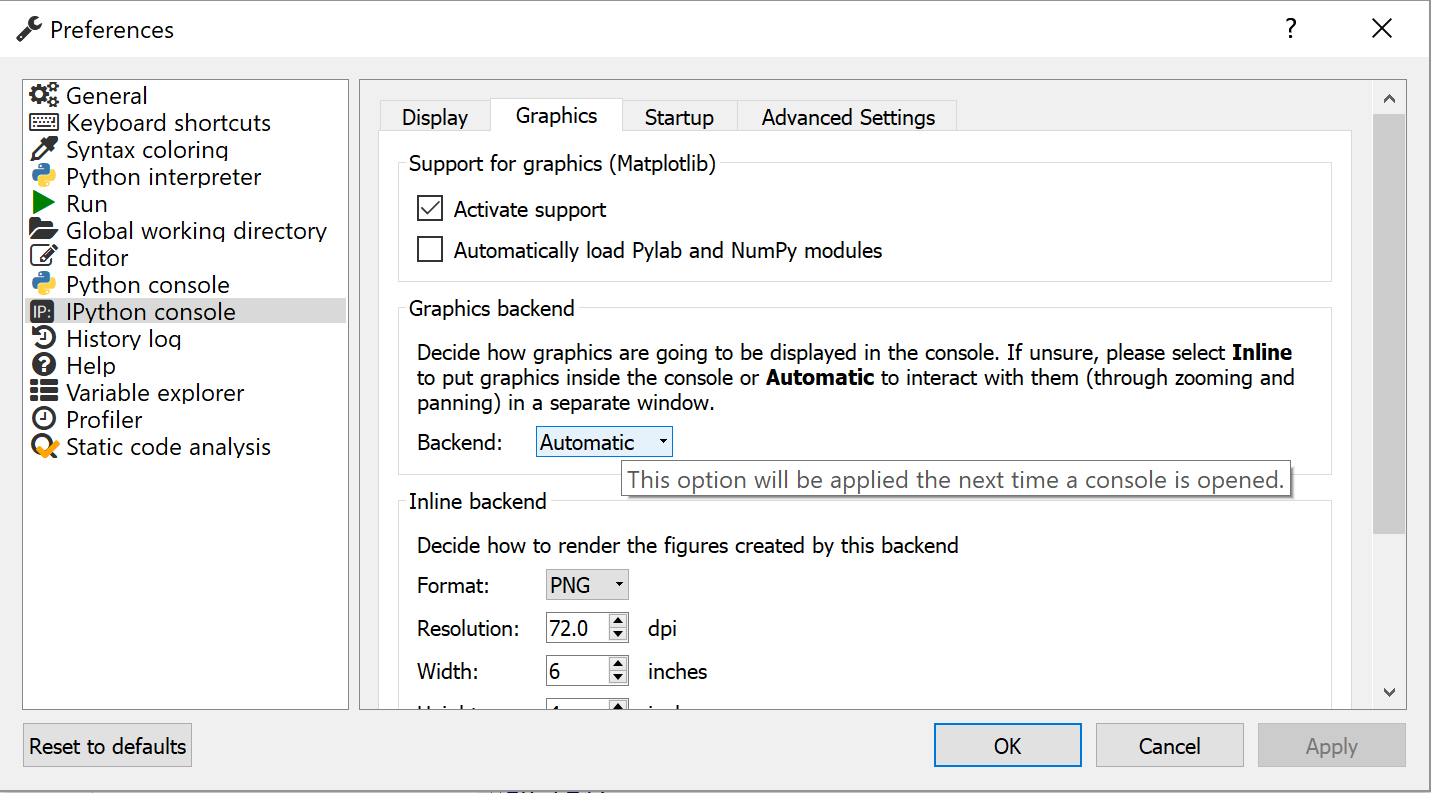• Q: How can I display real-time plots in Jupyter Notebooks?
• A: Add the following code to allow real-time plots to display instead of creating a new plot for every frame. Use the clear_output() function in the loop where the plot is displayed.
from IPython import display

display.clear_output(wait=True)

Here is a complete example:

import numpy as np
import matplotlib.pyplot as plt
from IPython import display

x = np.linspace(0, 6 * np.pi, 100)
cos_values = []
sin_values = []
for i in range(len(x)):
# add the sin and cos of x at index i to the lists
cos_values.append(np.cos(x[i]))
sin_values.append(np.sin(x[i]))

# graph up until index i
plt.plot(x[:i], cos_values[:i], label='Cos')
plt.plot(x[:i], sin_values[:i], label='Sin')
plt.xlabel('input')
plt.ylabel('output')

# lines that help with animation
display.clear_output(wait=True)  # replace figure
plt.pause(.01)  # wait between figures

• Q: How can I use the other pins on the Arduino if I'd like to add an additional sensor or actuator?
• A: The firmware that comes pre-loaded (tclab.ino) is specific to this lab with 2 heaters, 2 temperature sensors, and 1 LED. A different firmware called Standard Firmata can be loaded onto the Arduino to allow the pyFirmata (Python) package to read from or write to any of the pins. This may be useful if you are adding additional sensors or want to modify the pin connections.

import time

try:
from pyfirmata import Arduino, util
except:
try:
from pip import main as pipmain
except:
from pip._internal import main as pipmain
pipmain(['install','pyfirmata'])
from pyfirmata import Arduino, util

# for MacOS or Linux
# install CH340G driver from https://goo.gl/pWMcU3
#board = Arduino('/dev/cu.wchusbserial1420')

# for Windows
board = Arduino('COM5')

# Start iterator
iterator = util.Iterator(board)
iterator.start()

# TMP36 Temperature Sensor Calibration
def TMP36(Tv):
return (Tv*5000.0-500.0)/10.0

# Read voltage (0-5 V) as (0-1)
Tv1 = board.get_pin('a:0:i')
Tv2 = board.get_pin('a:2:i')

# Setup Temperature functions
def T1():

def T2():

# Setup Heater functions with PWM
H1 = board.get_pin('d:3:p')
H2 = board.get_pin('d:5:p')

time.sleep(1.0)

# Print Temperature 1
print('Temperature 1: ' + str(T1()))
print('Temperature 2: ' + str(T2()))

# Turn on heaters
print('Heater 1: 90% and Heater 2: 20%')
H1.write(0.9)
H2.write(0.2)

# Wait
print('Sleep for 25 seconds')
time.sleep(25.0)

# Print Temperatures 1 and 2
print('Temperature 1: ' + str(T1()))
print('Temperature 2: ' + str(T2()))

# Turn off heaters
H1.write(0.0)
H2.write(0.0)

board.exit()